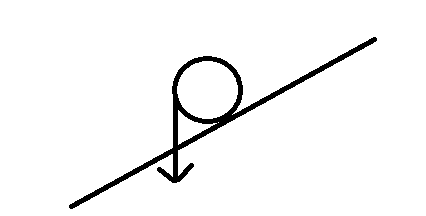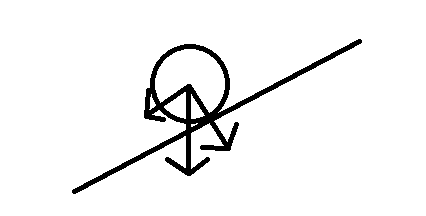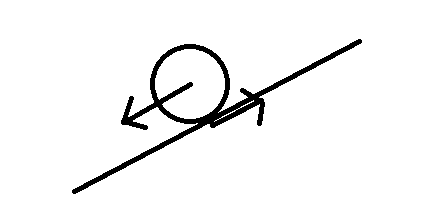# Which will reach the bottom first?

hello

let's say we have two cylindrical rodes of the same material and of the same length, but with different diameters

we place them in an inclined floor and let them roll without friction

which will reach the bottom first? the thick or the thin?

my understanding for this question is that we need to calculate the torque that makes the rod roll, which is depended on the weight of the rod and the diameter of the rod

then we need to calculate the angular velocity that this torque generates, which is depended on the circumference of each rod, along with the torque generated due to the mass/weight of each rod

in my opinion, we cannot be sure that one or the other will move faster, if we don't do these calculations

or I miss something?

thanks!

Svein
we place them in an inclined floor and let them roll without friction
If there is no friction, why would they roll?

•davenn and billy_joule
ok, then only air resistance is negligible

If there is no friction, why would they roll?

so without friction, they would be static? what about the torque generated by their weight? it would cause them to rotate endlessly without moving out of their position at all?

Last edited:
jbriggs444
Homework Helper
so without friction, they would be static? what about the torque generated by their weight?
If you draw a free body diagram containing only the normal force and the force of gravity but no friction, where do you see a torque?

Edit: In particular, a torque about the center of the cylinder.

If you draw a free body diagram containing only the normal force and the force of gravity but no friction, where do you see a torque?

Edit: In particular, a torque about the center of the cylinder.so what is your opinion? that the rod will stay there immobilized?

jbriggs444
Homework Helper
Why do you draw the force of gravity as if it acts on the left edge of the cylinder?

you can have it like that, but don't make the mistake to replace the whole rod with a point bodythere is still a couple of forces exerted, which will make the rod to roll, given there is rolling friction, but not air resistancejbriggs444
Homework Helper
I see a "free body diagram" with a force of gravity correctly positioned to act at the center of gravity of the cylinder but with two additional force vectors at right angles. What are those? And where is the normal force from the slope on the cylinder?

I see a free body diagram with a force acting parallel to the downward slope acting on the center of the cylinder and a force in the opposite direction acting at the point of contact. What force is represented by the down-slope arrow? What force is represented by the up-slope arrow?

A.T.
there is still a couple of forces exerted, which will make the rod to roll, given there is rolling friction, but not air resistance
Do you mean there is static contact friction that makes it roll, but no rolling resistance that dissipates energy? If that's the case, then you can use energy conservation to find the speeds.

Do you mean there is static contact friction that makes it roll, but no rolling resistance that dissipates energy? If that's the case, then you can use energy conservation to find the speeds.

exactly
so, from energy conservation, we deduct that the larger mass will have the (much) lower velocity
but, the potential energy is not quite the same at the begining, because the center of mass is a big higher in the larger mass
so are there couples of cylinders (with different diameter) that the larger will be slower and other couples where the larger will be faster?

I see a "free body diagram" with a force of gravity correctly positioned to act at the center of gravity of the cylinder but with two additional force vectors at right angles. What are those? And where is the normal force from the slope on the cylinder?

I see a free body diagram with a force acting parallel to the downward slope acting on the center of the cylinder and a force in the opposite direction acting at the point of contact. What force is represented by the down-slope arrow? What force is represented by the up-slope arrow?

you see the analysis of the force of gravity
and in the second, you see the moving part of the weight force analysis, and its couple force at the point of friction

A.T.
the center of mass is a big higher in the larger mass
The change in height is the same for both cylinders, and the change in potential energy is all that matters.

jbriggs444
Homework Helper
you see the analysis of the force of gravity
and in the second, you see the moving part of the weight force analysis, and its couple force at the point of friction
Note that the force of gravity produces zero torque about the center of cylinder. The only force that produces a non-zero torque is the force of friction at the point of contact.

Note that there is no guarantee that the force of friction is equal in magnitude to the resultant of gravity plus the normal force. To my mind, that means that referring to the two as a "couple" is not accurate.

jbriggs444
Homework Helper
The point is not to give you the answer. The point is to get you to reason out the answer for yourself.

I found the answer: the bigger mass the lowest the velocity, is this the case?

jbriggs444
Homework Helper
No

are they reaching the bottom the same time?

jbriggs444
Homework Helper
Please give your reasoning based on "energy conservation" that the velocity of the bigger cylinder is smaller.

difference in potential energy from top to botton is bigger for the larger mass, since height and gravity acceleration are the same

so difference in kinetic energy from top standing point until bottom is bigger in larger mass

but we cannot deduce about the ending velocities

jbriggs444
Homework Helper
The final energies (rotational and kinetic) can be calculated in terms of the final velocity. Do you know the formulas for rotational kinetic energy and linear kinetic energy as a function of velocity?

no, I only know linear which is 1/2mV^2

jbriggs444
Homework Helper
The formula for rotational energy is analogous -- it is ##\frac{1}{2}I\omega^2## where ##I## is the "moment of inertia" of the object and ##\omega## is its rotation rate, also known as "angular velocity". The angular velocity is normally measured in radians per second (this makes the units come out nice). For a given shape, the moment of inertia is proportional to its mass and is proportional to the square of its size.

If you work out the proportions, a cylinder of a given material rolling at a fixed velocity whose radius is doubled...

1. Rotates half as fast due to the increased radius and has 1/4 of the kinetic energy due to that reduced rotation rate.
2. Has four times the moment of inertia due to the increased radius and 4 times the kinetic energy due to that increased moment of inertia.
3. Has four times the mass due to the increased radius and has 4 times the kinetic energy due to that increase mass.

The net increase in rotational kinetic energy is a factor of four. That exactly matches the increase in mass. Just like the increase in linear kinetic energy exactly matches the increase in mass. The two remain proportional to one another!

[Edit, first draft of this missed the increase in moment of inertia]